# The Gentlest Introduction to Tensorflow – Part 3

This post is the third entry in a series dedicated to introducing newcomers to TensorFlow in the gentlest possible manner. This entry progresses to multi-feature linear regression.

By Soon Hin Khor, Co-organizer for Tokyo Tensorflow Meetup.

Editor's note: You may want to check out part 1 and part 2 of this tutorial before proceeding.

### Quick Review

The premise of the previous articles was: given any house size (square meters/sqm), which is the feature, we want to predict the house price (\$), the outcome. To do that we:

1. We find a straight line (linear regression) that ‘best-fits’ the data points that we have. The ‘best-fit’ is when the linear regression line ensures that the difference between the actual data points (gray dots) and the predicted values (gray dots interpolated on to the straight line), which, in other words, is the sum of multiple blue lines, is minimized.
2. With this straight line we can predict any value of house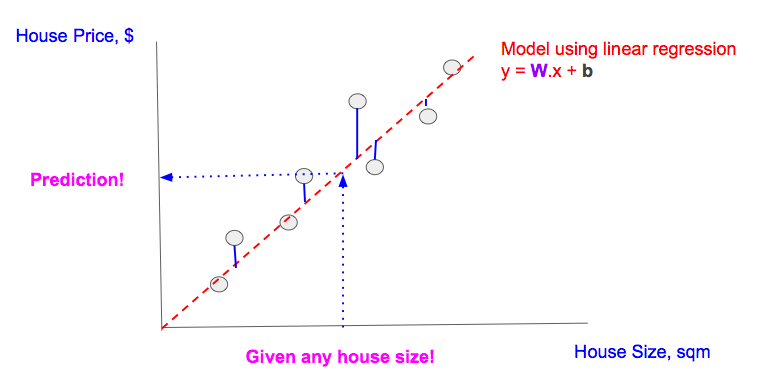Predicting using Single-feature Linear Regression.

### Multi-feature Linear Regression Overview

In reality, any prediction relies on multiple features, so we advance from single-feature to 2-feature linear regression; we chose 2 features to keep visualization and comprehension simple, but the concept generalizes to any number of features.

We introduce a new feature, ‘Rooms’ (number of units in the house). When collecting datapoints, we must now collect values for the new feature ‘rooms’ on top of the existing feature ‘house size’, as well as the corresponding outcome ‘house price’.
Our chart becomes 3-dimensional.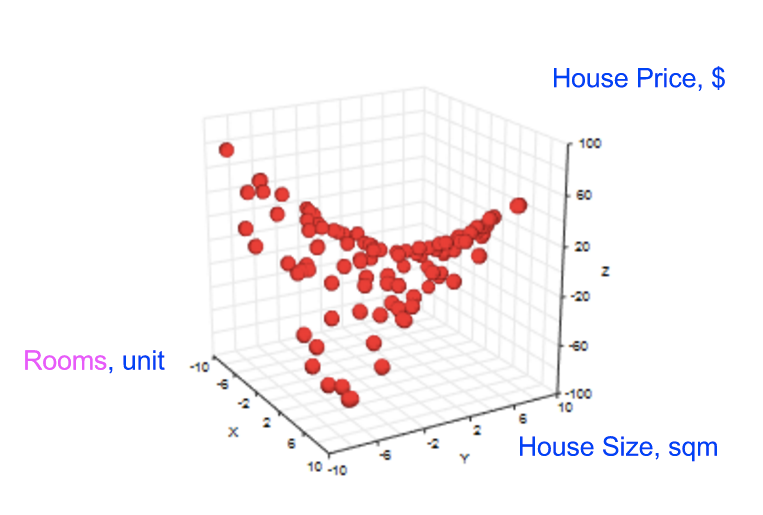Datapoints for the outcome ‘House Price’ and its 2-feature (‘Rooms’ & ‘House Size’) space.

Our goal then becomes predicting ‘house price’, given ‘rooms’, and ‘house size’ (see image below).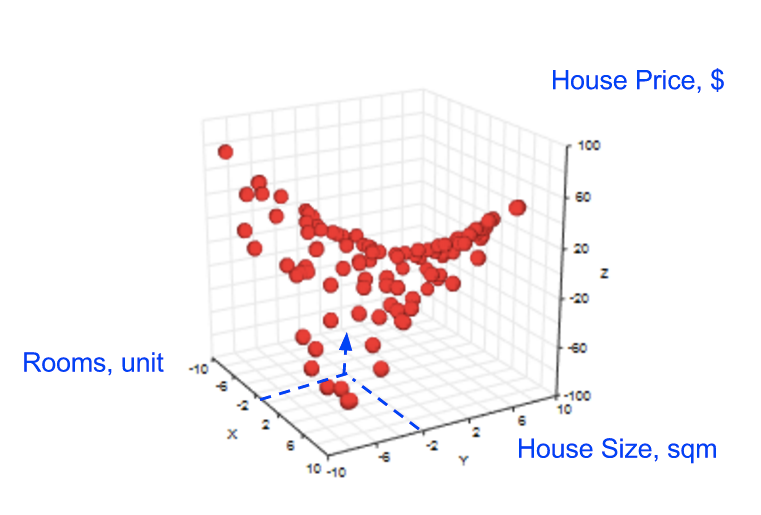Prediction for a given 2-feature sometimes cannot be done due to missing of datapoints.

In the single-feature scenario, we had to use linear regression to create a straight line to help us predict the outcome ‘house size’, for cases where we did not have datapoints. In a 2-feature scenario, we can also employ linear regression, but to create a plane (instead of a straight line) to help us predict (see image below).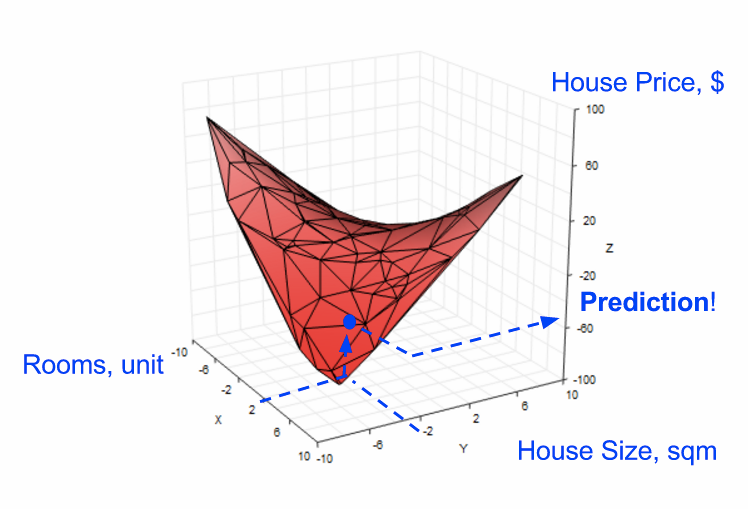Using linear regression on 2-feature space to create a plane to do prediction.

### Multi-feature Linear Regression Model

Recall for a single-feature (see left of image below), the linear regression model outcome (y) has a weight (W), a placeholder (x) for the ‘house size’ feature, and a bias (b).

For 2-feature (see right of image below), we introduce another weight, which we call W2, and another placeholder, x2 to hold the ‘rooms feature value.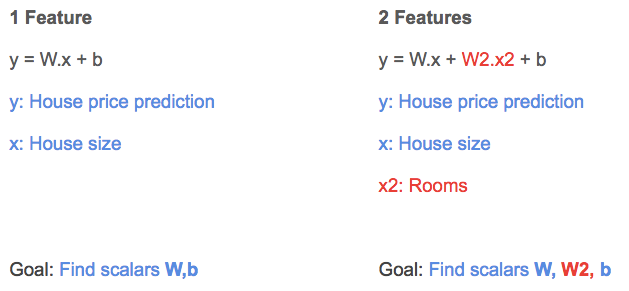1-feature vs. 2-feature linear regression equations.

When we perform linear regression, gradient descent helps us learn the additional weight W2, on top of the learning W, b as previously discussed.

### Multi-feature Linear Regression in Tensorflow

Quick Review
Our TF code for single-feature linear regression consists of 3 parts (see image below):

• Constructing the model (blue part)
• Constructing the cost function based on the model (red part)
• Minimizing the cost function using gradient descent (green part)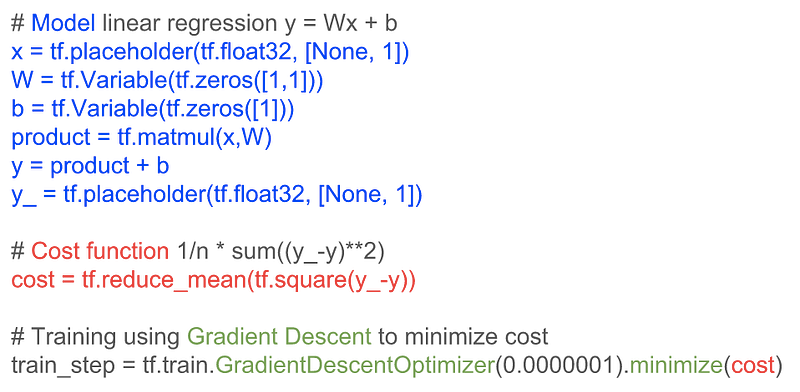Tensorflow code for 1-feature linear regression.

Tensorflow for 2-feature Linear Regression

The change to support 2-feature linear regression equation (explained above) in TF code is shown in red.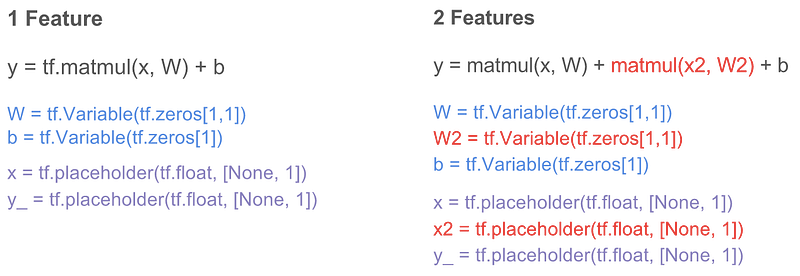Note this way of adding new features is inefficient; as the number of features grow, the number of required variables and placeholders increases. In reality models have many more features, which worsens this problem. How can we represent features efficiently?

### Matrices to the Rescue

First, let us generalize representing a 2-feature model to an n-feature one: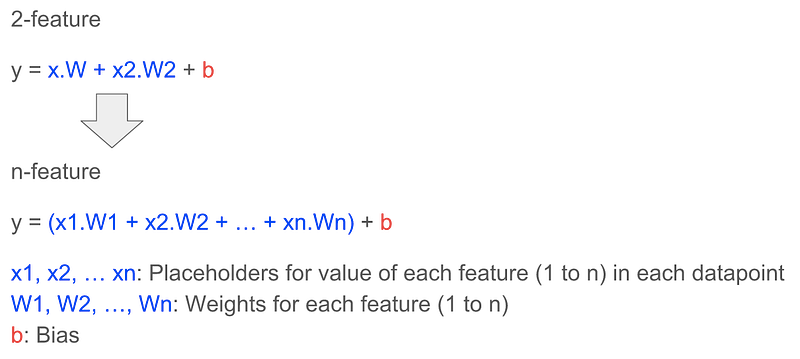It turns out that the complex n-feature formula can be simplified in the world of matrices, and matrices are in-built into TF for these reasons:

• Data can be represented in multi-dimensions, which fits the way we want to represent a datapoint with n features (below left, also known as the feature matrix) and a model with n weights (below right, also known as the weight matrix)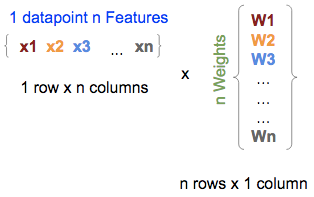1 datapoint’s n Features and the model’s n Weights in matrix form.

In TF, they would be written as:

``````
x = tf.placeholder(tf.float, [1,n])

W = tf.Variable(tf.zeros[n,1])
``````

NOTE: For W we use tf.zeros, which initializes all W1, W2, ..., Wn to zeros.

• Mathematically matrix multiplication is a sum of multiplications (just accept this as part of mathematics); thus naturally the matrix multiplication between the features (the one in the middle) and weights (the one on the right) matrices gives you the outcome (the one on the left), which is equivalent to first part of the n-feature linear regression formula (described above), i.e., without the biases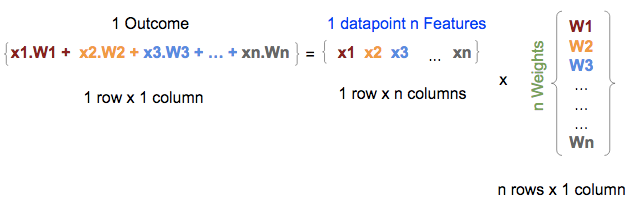Matrix multiplication between Features and Weights matrices gives the outcome (without biases added).

In TF, this multiplication would be:

``````
y = tf.matmul(x, W)
``````

• Matrix multiplication between a multi-row feature matrix (each row representing a datapoint’s n features), returns multi-row outcomes (each row representing the outcome/prediction (without bias added) of each datapoint); thus a single matrix multiplication can apply the linear regression formula to multiple datapoints to produce multiple predictions, one for each datapoints, at a single go (see below)!

Note: The x representations in the feature matrix become more complex, i.e., we use x1.1, x1.2, instead of x1, x2, etc. because the feature matrix (the one in the middle) has expanded from representing a single datapoint of n-features (1 row x n columns) to representing m datapoints with n-features (m rows x n columns), so we extended x<n>, e.g., x1, to x<m>.<n>, e.g., x1.1, where n is the feature number and m is the datapoint number.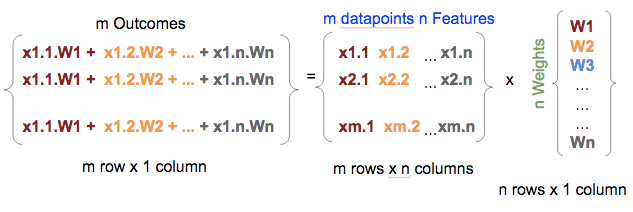Multiple row matrix multiplication with model weights produce multiple row matrix outcomes.

In TF, they would be written as:

``````
x = tf.placeholder(tf.float, [m, n])

W = tf.Variable(tf.zeros[n,1])

y = tf.matmul(x, W)
``````

• Finally, adding a constant to the outcome matrix results in the constant being added to every row in the matrix

In TF, with our x, and W represented in matrices, regardless of the number of features our model has or the number of datapoints we want to handle, it can be simplified to:

``````
b = tf.Variable(tf.zeros)

y = tf.matmul(x, W) + b
``````

### Tensorflow Multi-feature Cheatsheet

We do a side-by-side comparison to summarize the change from single to multi-feature linear regression: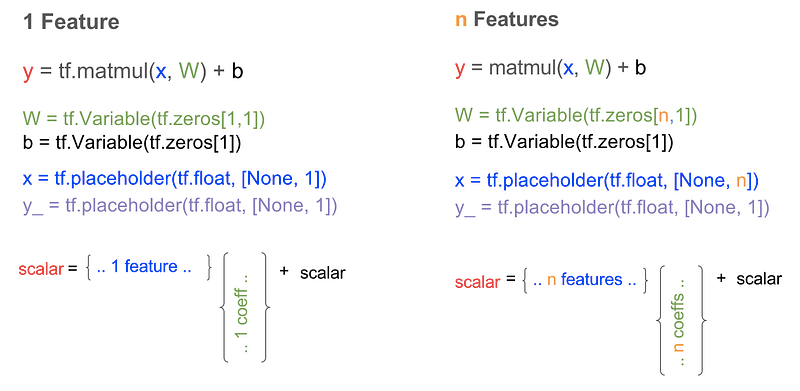1-feature vs n-feature linear regression model in Tensorflow.

### Wrapping Up

We illustrated the concept of multi-feature linear regression, and showed how we extend our model and TF code from single to 2-feature linear regression models, which is generalizable to n-feature models. We conclude by presenting a cheatsheet for multi-feature TF linear regression model.

Coming Up Next

We will present the concepts of logistic regression, cross-entropy, and softmax, which will enable us to fully understand Tensorflow’s official beginner’s tutorial on MNIST.

### References

Bio: Soon Hin Khor, Ph.D is using tech to make the world more caring, and responsible. Contributor of ruby-tensorflow. Co-organizer for Tokyo Tensorflow meetup.

Original. Reposted with permission.

Related: